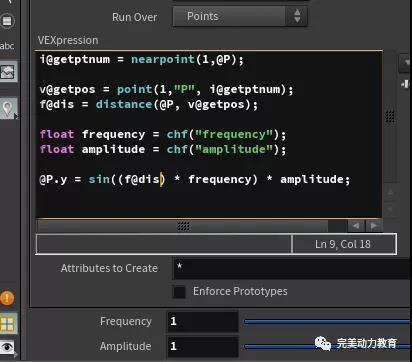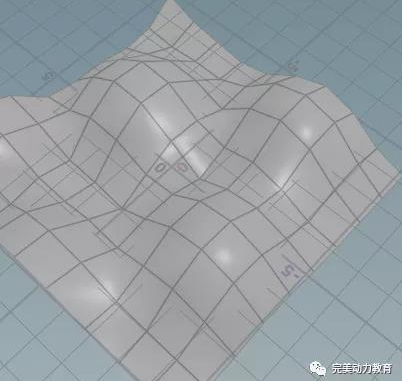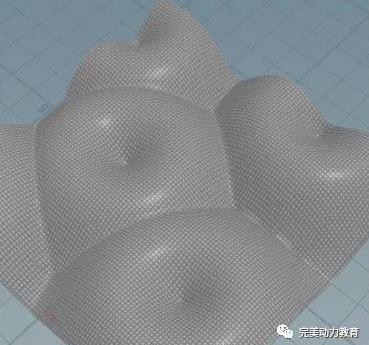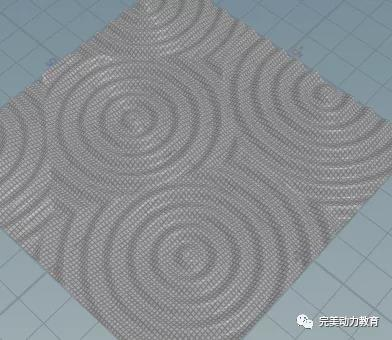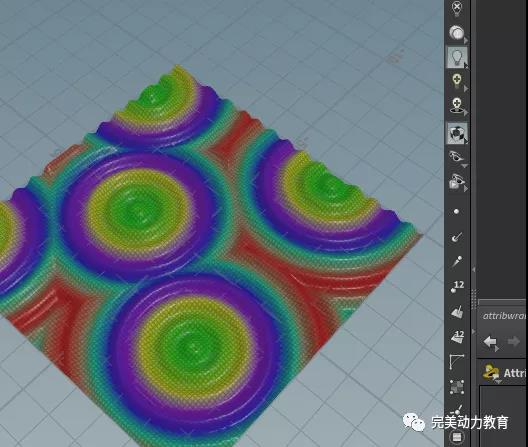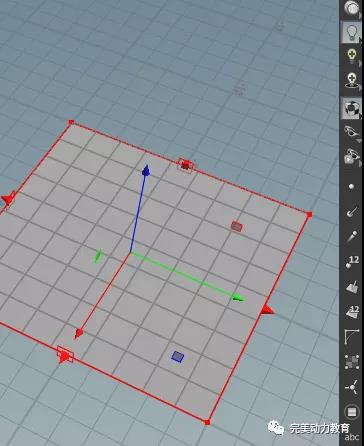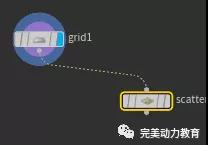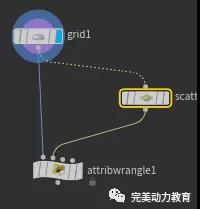i@getptnum = nearpoint(1,@P)；

v@getpos = point(1,"P", i@getptnum)；

f@dis = distance(@P, v@getpos)；

float frequency = chf("frequency")；

float amplitude = chf("amplitude")；

@P.y = sin((f@dis) * frequency) * amplitude；